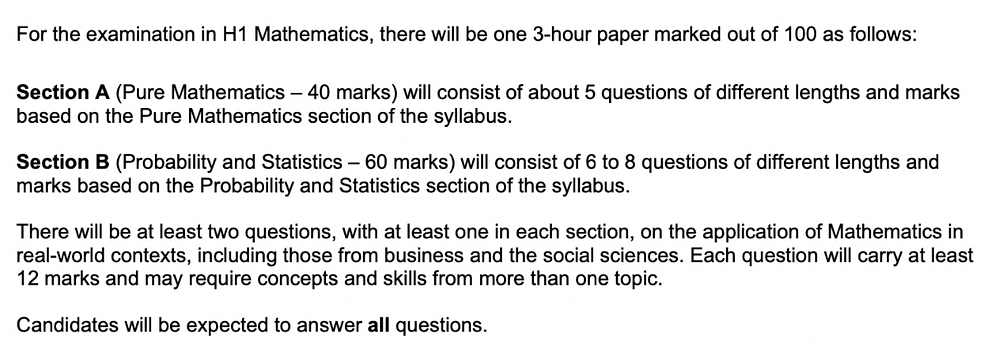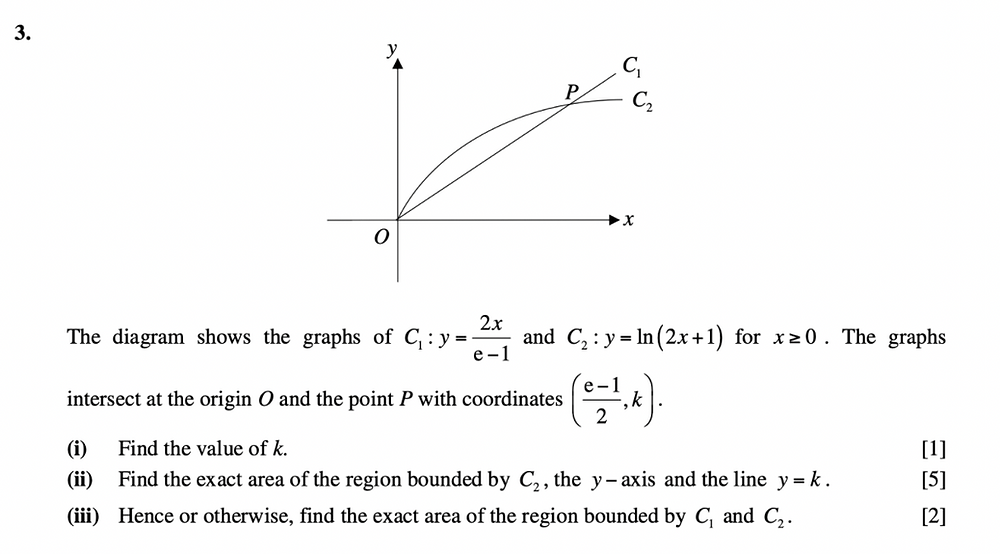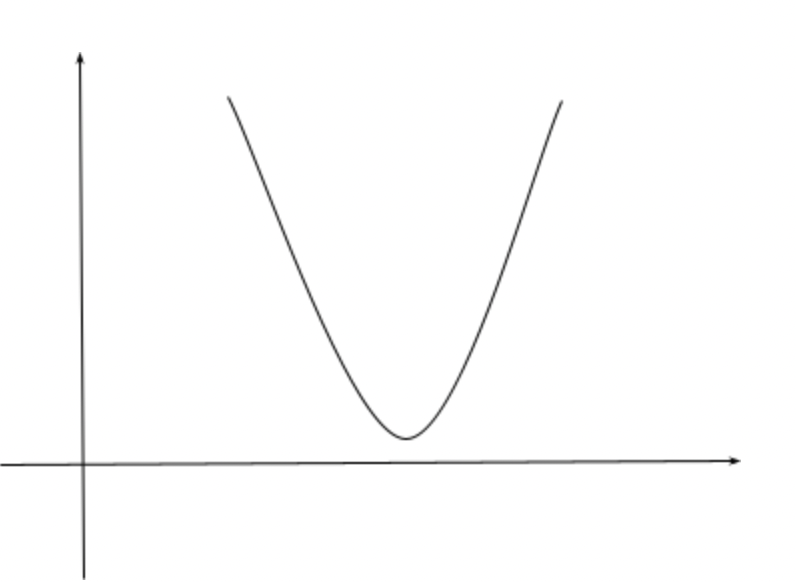Search
•Zenith Education Studio

# How To Ace your A Level H1 Mathematics Examinations (Section A)As Albert Einstein once said, “Pure Mathematics is, in its way, the poetry of logical ideas”. At Zenith, we strongly believe that the study of Mathematics is for and can be appreciated by everyone. Some students walk in claiming, “No way, I’m just not a numbers person”, but grow to love the subject and perform excellently during their A Level Mathematics examinations. This article is designed to be easily digestible with practical steps that you can apply without difficulty; it aims to teach you how to ace Section A of the A Level H1 Mathematics Examination!

First, let’s take a look at how the A Level H1 Mathematics syllabus is structured. The A Level H1 Mathematics syllabus consists of only one 3-hour exam paper. It is graded out of 100 marks, but it is nonetheless split into two different sections, just like the A Level H2 Mathematics paper. As shown in Fig 1., Section A (40 marks) covers questions on Pure Math while Section B (60 marks) covers questions on Probability and Statistics. While this mark allocation means that a larger percentage of your grade at the A Level H1 Mathematics examinations is dependent on your grasp of concepts under Section B, getting that much-coveted ‘A’ grade will require you to do well for Section A too! Check out our article on Section B: Probability and Statistics here!Fig 1. Breakdown of the A Level H1 Mathematics Examination paper

Here are the topics that are tested during the A Level H1 Mathematics examinations under Pure Math in Section A:

• Functions and Graphs

• Exponential and logarithmic functions and graphing techniques

• Equations and inequalities

• Calculus

• Differentiation

• Integration

Pure Math is often perceived as more challenging for H1 Mathematics students as it covers abstract concepts which require students to be well-acquainted with various Mathematical proofs. In particular, students may find application questions confusing, as it can be difficult to envision how abstract Mathematical theories are relevant to everyday life. This is why, to help you achieve the grades you desire, Zenith, Singapore’s top A Level Mathematics tuition center, is looking to share the top 5 important things to take note of for Section A (Pure Math) of the A Level H1 Mathematics Examinations.

#1: Make sure you understand how to solve for integrals as the area under a graph.

In graphical format, integrating a Mathematical equation provides you with the area underneath the graph of the equation, as bounded by the x and y-axes, or by another graph. Questions demanding students to find the area of the region bounded by the axes and a graph are common at the A Level H1 Mathematics Examinations. For instance, you can refer to Fig 2. below, where Questions 3 (ii) and (iii) require students to find the areas of the region bounded by the various axes and graphs as shown in the diagram. It is important to note that when a question asks for the exact area, you are not allowed to use your GC and present the answer correct to 3 significant figures.Fig. 2 Question 3 from Catholic Junior College’s 2018 A Level H1 Mathematics Prelims

To tackle parts (i) and (ii), students have to first find the point of intersection between the two graphs, at the point P. The x-coordinate of P has been provided in the question and students only need to figure out what k represents, which will give them 1 mark as indicated by part (i). To do this, you simply have to sub the equation of either C1 or C2 withand solve it for k. For part (ii), it is important to note that the question asked for the region bounded by the y-axis, which means that integration has to be done with respect to y. We are hence doing dy instead of dx. Therefore, since the equations of the graphs were provided in terms of x, students have to change the subject such that the equations are in terms of y. What you will get is that the equation in terms of y isThereafter, you should change the subject of the equation to x, and integrate the equation of C2 with the upper limit as k and with the lower limit as 0, as C2 intersects the y-axis at the origin. This provides them with the exact area of the region bounded by C2, the y-axis and y=k.

For (iii), students are expected to find the exact area of the region bounded by the two graphs. If you try to visualize this, it points to the area bounded by C1 and C2. To calculate the exact area, you first have to find the area bounded by C2 and the x-axis. Thereafter you should find the exact area bounded by C1 and the x-axis. Next, minus the area under the graph of C2 from the area under the graph of C1. However, as this question states that this can be done “hence or otherwise” (referencing part (ii) of the question), it means that part (ii) provided us with a “shortcut” for completing the question. There is hence no need to conduct integration again. This is also why the question is only worth 2 marks, while part (ii) is worth 5 marks.

#2: Always remember that every input belonging to a function can only have one output.

Students often forget that a function is a special type of Mathematical relation where each input can only have one output. Let’s take f(x) = x^3 as an example. When we input x = 3 into f(x) = x^3, the answer that we get is f(3) = 3^3 = 27. It is not possible for us to get another answer other than 27.

During the A Level H1 Mathematics Examinations, the function questions asked are, of course, more complicated than the above example. For instance, you might get f(x) = x^3 + 2x - 5. Some of these equations, when subbed and solved for, especially in graphical form, which is part of the A Level H1 Mathematics syllabus, might appear to provide you with more than one output. You should always check to accept only the correct output and reject the others. Some functions provide you with a range of x, for instance, that x > 0, therefore, the outputs of f(x) when x ≤ 0 should be rejected. In graphical form, the entire portion of the graph where x ≤ 0 is invalid.

#3: Apply the correct rules for Differentiation

You are only allowed to split an equation in the situation where the two expressions are supposed to be multiplied with or divided by each other, as in the following examples:A shorter method, however, would be expanding the equation and solving it directly, as in the following:As such, during the H1 A Level Mathematics Examinations, you should be very clear of when you can or cannot expand a particular mathematical expression, and of the various rules you can use for Differentiation! This will prevent you from making unnecessary errors, which can save you many marks since a wrong expansion usually happens in the first step, resulting in you getting 0 marks for the entire question.

#4: Make sure you know the conditions for ax^2 + bx + c to be always positive (or always negative).

This is an important concept that you will need to know for inequalities, graphs and functions. Questions might also ask you to find the value of x such that ax^2 + bx + c is always positive or always negative, which means that you need to be aware of what the phrase denotes.

ax^2 + bx + c is always positive when no real roots exist, and a is positive. The graph of the polynomial, as shown in Fig 3., will always lie above the x-axis (i.e. the graph of ax^2 + bx + c , when always positive, will not intersect the x-axis at any point). If you are solving for the range of values for the unknowns a, b, and c, you should solve for b2 - 4ac < 0.Fig 3. Example of a polynomial that is always positive (lying above the x-axis)

Contrastingly, ax^2 + bx + c is always negative when no real roots exist, and a is negative. The graph of the polynomial, as shown in Fig 4., will always lie below the x-axis (i.e. the graph of ax^2 + bx + c , when always negative, will not intersect the x-axis at any point). If you are solving for the range of values for the unknowns a, b, and c, you should solve for b^2 - 4ac > 0. To make it easier to remember, positive graphs are normally in a "smiley face" formation as per Fig 3., while a negative graph would take on the "frowning face" formation, as per Fig 4.Fig 4. Example of a polynomial that is always negative (lying below the x-axis)

Make sure that you always use the correct Mathematical notations.

Students sometimes get confused over when to use certain Mathematical notations. For instance, some might make mistakes between > and ≥, or ≤ and <. To clarify, > means greater than, while ≥ means greater than or equals to. The opposite applies for smaller than and smaller than or equals to. Scroll down to pages 9-13 of the syllabus, which can be accessed here, for the Mathematical notations typical of the A Level H1 Mathematics examinations. Questions in the A Level H1 Mathematics examinations might use any of these notations, and you are expected to understand and be able to use them in your own workings.

Always round off your answers to the correct number of significant figures (s.f.).

Again, this is an easy mistake to fix. It is standard practice that, when doing your working, students leave their answers to 5 or 6 significant figures. This is to prevent premature rounding which can make your final answer inaccurate. The final answer is typically to be presented correct to 3 significant figures, or 1 decimal place for angles in degrees. If the question specifies a different level of accuracy, follow that instead.

Some questions at the A Level H1 Mathematics examinations demand you to present your answers without the use of a calculator (in simplified form) However, nobody said that you cannot check your answers using your GC. Using your GC to check is easy to execute and helps you to confirm that you have not made any errors in your manual calculations.

With that, Zenith has come to the end of this article, with 5 useful tips on how to perform your best for Section A of the A Level H1 Mathematics Examinations. In sum, this article has taught you to:

• Being able to solve for the area of a region using Integration is important

• Functions can only have one output for each input

• Know the right Mathematical notations to avoid misunderstanding questions

• Present your answers to the correct number of significant figures to avoid inaccurate answers which results in a loss of marks

Looking for more tips and tricks to score at the A Level H1 Mathematics Examinations? Find out more by reading this article!

At Zenith, we understand that preparing for H1 Mathematics examinations can be extremely stressful. This is why our young yet experienced tutors strive to make lessons fun, interesting and engaging. As Edsger W. Dikjstra, a Dutch Systems Scientist once said, “There should be no such thing as boring Mathematics.” While ensuring our students have an enjoyable time in class, Zenith boasts a stellar A-rate of nearly 2x the national average, and are always looking to help more students reach their fullest potential! Find out more about our meticulously curated A Level H1 Mathematics tuition programme here, and sign up for a free trial lesson today! Bring a friend or two––we look forward to seeing you in class, the more the merrier!